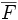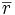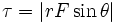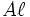# Difference between revisions of "Torque"

## Definition

### Due to a single force, about a point

Suppose$\overline{F}$ is a force and$\overline{r}$ is the radial vector from a point$A$ to the point of application of$\overline{F}$. The torque due to$\overline{F}$ about$A$ is defined as the cross product of$\overline{r}$ and$\overline{F}$. In other words, it is defined as:$\overline{\tau} = \overline{r} \times \overline{F}$

The torque is a vector quantity. (More properly, torque is an alternating 2-tensor, and is treated as a vector via a (non-canonical) identification of$\R^3$ with the space of alternating 2-tensors on$\R^3$).

The magnitude of torque is given by:$\tau = |rF \sin \theta|$

where$\theta$ is the angle between the radial vector and the force vector.

Note that the torque, as a vector quantity, depends only on the point$A$, the line of action of$\overline{F}$, and the magnitude of$\overline{F}$. Thus, two forces of the same magnitude with the same line of action (Even though they may act at different points) generate the same torque.

### Due to a single force, about an axis

Suppose$\overline{F}$ is a force and$\overline{r}$ is the vector from (and perpendicular to) a line$\ell$ to the point of application of$\overline{F}$. The torque due to$\overline{F}$ about$A\ell$ is defined as the cross product of$\overline{r}$ and$\overline{F}$. In other words, it is defined as:$\overline{\tau} = \overline{r} \times \overline{F}$

The torque is a vector quantity. (More properly, torque is an alternating 2-tensor, and is treated as a vector via a (non-canonical) identification of$\R^3$ with the space of alternating 2-tensors on$\R^3$).

The magnitude of torque is given by:$\tau = |rF \sin \theta|$

where$\theta$ is the angle between the radial vector and the force vector.

### Due to multiple forces

The torque doe to multiple forces is defined as the vector sum of the torques due to each of the forces. When there is a continuum of forces, it is defined as a suitable integral. Fill this in later

## Units and dimensions

The dimensions of torque are